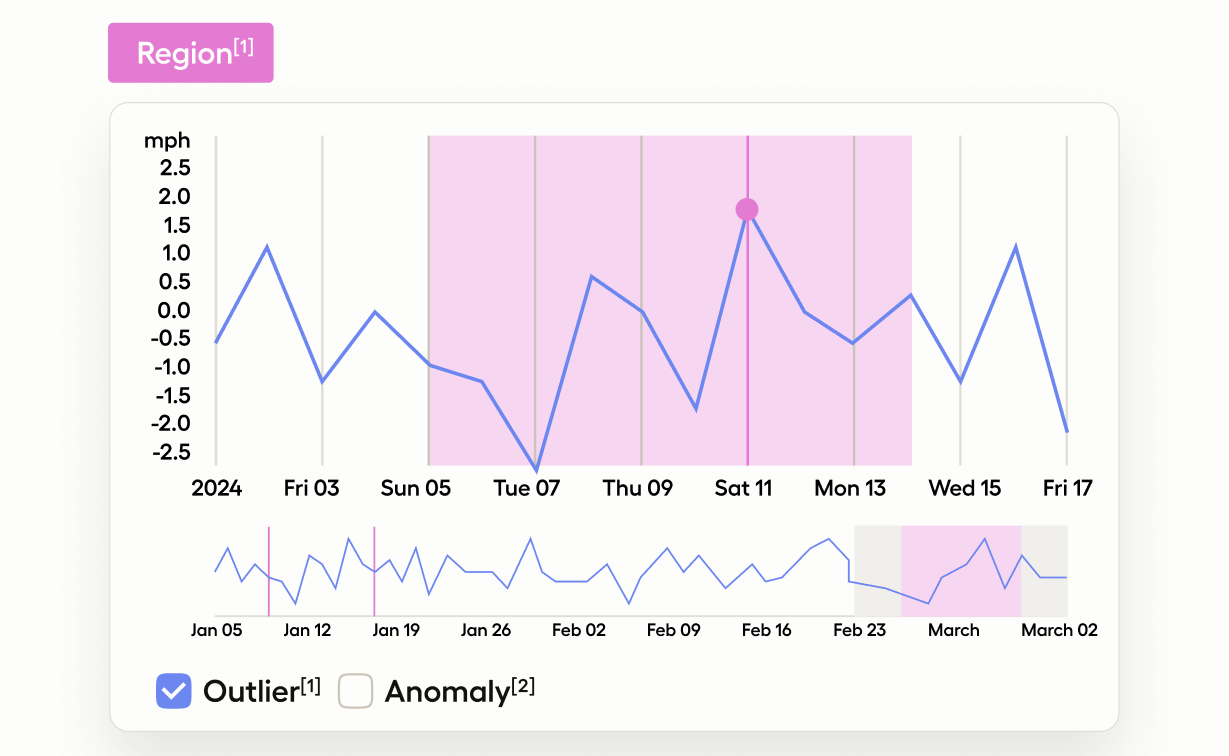# Outliers and Anomaly DetectionIf you want to train a machine learning model to detect outliers and anomalies in time series data, use this template to label suspicious regions and classify those regions of the time series channels as outliers or anomalies.

## Labeling Configuration

``````<View>

<TimeSeries name="ts" valueType="url" value="\$csv"
sep=","
timeColumn="time"
timeFormat="%Y-%m-%d %H:%M:%S.%f"
timeDisplayFormat="%Y-%m-%d"
overviewChannels="velocity">

<Channel column="velocity"
units="miles/h"
displayFormat=",.1f"
strokeColor="#1f77b4"
legend="Velocity"/>
</TimeSeries>
<TimeSeriesLabels name="label" toName="ts">
<Label value="Region" background="red" />
</TimeSeriesLabels>
<Choices name="region_type" toName="ts"
perRegion="true" required="true">
<Choice value="Outlier"/>
<Choice value="Anomaly"/>
</Choices>
</View>``````

All labeling configurations must be wrapped in View tags.

Use the TimeSeries object tag to specify the time series data source. The `valueType="url"` parameter specifies that the time series data is available as a URL, rather than a file, and the `value="\$csv"` parameter specifies that the URL is stored in a data key called `csv`. The `sep=","` parameter specifies that a comma is the data separator, as is standard for a CSV-formatted file. The time parameters specify which column contains the time data, the format of the time data in the file, and how to display the time data on the labeling interface.

``````<TimeSeries name="ts" valueType="url" value="\$csv"
sep=","
timeColumn="time"
timeFormat="%Y-%m-%d %H:%M:%S.%f"
timeDisplayFormat="%Y-%m-%d"
overviewChannels="velocity">
<Channel column="velocity"
units="miles/h"
displayFormat=",.1f"
strokeColor="#1f77b4"
legend="Velocity"/>
</TimeSeries>``````

The `overviewChannels` parameter in the TimeSeries tag specifies the column in the time series data to display on the time series graph as a channel. You can then use the Channel tag and its parameters to provide details about the data, such as the units of the data, the `displayFormat` of the channel data using d3 format, the `strokeColor` to use when highlighting the channel, and the name of the channel as it should appear in the labeling interface legend.

Use the TimeSeriesLabels control tag to specify the labels to apply to regions in the time series graph:

``````<TimeSeriesLabels name="label" toName="ts">
<Label value="Region" background="red" />
</TimeSeriesLabels>``````

Use the Choices control tag to classify whether each specific region is an Outlier or an Anomaly in the time series data:

``````<Choices name="region_type" toName="ts"
perRegion="true" required="true">
<Choice value="Outlier"/>
<Choice value="Anomaly"/>
</Choices>``````